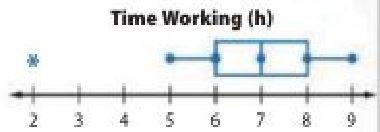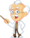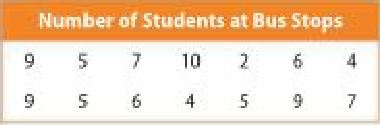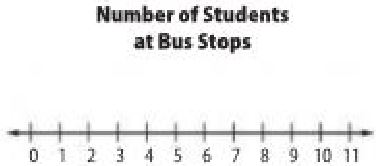Homework Explained - Math Practice 101Dear guest, you are not a registered member. As a guest, you only have read-only access to our books, tests and other practice materials.

As a registered member you can:

 Power Up     Test Practice Question 11 The box plot shows the number of hours spent working on a science project by students. Determine if each statement is a valid conclusion based on the box plot. Select yes or no.a. The distribution is symmetric. b. The median is the best measure to describe the center. c. There is an outlier at 2. No Yes Yes(show solution) Question 12 The list of data shows the number of students at different bus stops on Mr. Carter's route. Construct a line plot of the data.Circle the correct term to make each statement true. a. The distribution is (symmetric, not symmetric). b. There is a (gap, cluster) between 4 and 7. c. The (mean, median) is the best measure to describe the center. Type below:(show solution) Spiral Review   Select an appropriate display for each situation. Choose from the list shown. Bar Graph          Line Graph Box Plot             Line PLot Circle Graph       Double Bar Graph Scatter Plot        Double Line Graph Histogram          Double Box Plot Question 13 the number of cell phone subscribers for the past 5 years Type below:(show solution) Question 14 point totals for the top 10 NASCAR drivers Type below:(show solution) Question 15 the portion of a family's budget assigned to each category Type below:(show solution) Question 16 the median of the exam scores for one class Type below:(show solution) Question 17 gas mileage for 2013 cars Type below:(show solution)